# KSEEB Solutions for Class 6 Maths Chapter 12 Ratio and Proportion Ex 12.2

Students can Download Chapter 12 Ratio and Proportion Ex 12.2 Questions and Answers, Notes Pdf, KSEEB Solutions for Class 6 Maths helps you to revise the complete Karnataka State Board Syllabus and score more marks in your examinations.

## Karnataka State Syllabus Class 6 Maths Chapter 12 Ratio and Proportion Ex 12.2

Question 1.
Determine if the following are in proportion.
a) 15, 45, 40, 120
Solution:Therefore, 15 : 45 = 40 : 120
Hence, these are in proportion

b) 33, 121, 9, 96Therefore 33 : 121 ≠ 9 : 96
Hence, these are not in proportion

c) 24, 28, 36, 48Therefore, 24 : 28 ≠ 36 : 48
Hence, these are not in proportiond) 32, 48, 70, 210Therefore 32 : 48 ≠ 70 : 210
Hence, these are not in proportion

e) 4, 6, 8, 12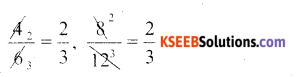Therefore 4 : 6 = 8 : 12
Hence, these are in proportion

f) 33, 44, 75, 100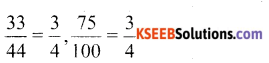Therefore, 33 : 44 = 75 : 100
Hence, these are in proportion

Question 2.
Write true (T) or False (F) against each of the following statement:
Solution:
a) 16 : 24 :: 20 : 30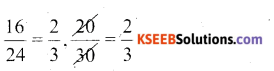Therefore 16 : 18 = 28 : 30
Hence, True

b) 21 : 6 :: 35 : 10Therefore 21 : 6 = 34 : 10
Hence, True

c) 12 : 18 :: 28 : 12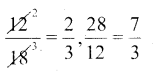Therefore 21 : 18 ≠ 28 : 12
Hence, Trued) 8 : 9 :: 24 : 27Therefore, True

e) 5 : 239 :: 3 : 4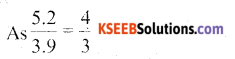Therefore 5.2 : 3.9 ≠ 3 : 4
Therefore 0.9 : 0.36 = 10 : 4
Hence, True

f) 0.9 : 0.36 :: 10.4Therefore 0.9 : 0.36 = 10.4
Hence, True

Question 3.
Are the following statements true?
Solution:
a) 40 persons : 200 persons = Rs 15 : Rs 75True

b) 7.5 litres : 15 litres = 5 kg : 10 kg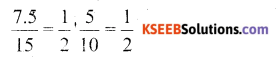True

c) 99 kg : 45 kg = Rs 44 : Rs 20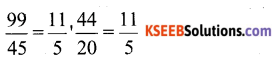True

d) 32 m : 64 m = 6 sec : 12 secTrue

e) 45km : 60 KM = 12 hrs : 15 hrs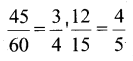FalseQuestion 4.
Determine if the following ratios from a proportion. Also, Write the middle terms and extreme terms where the ratios from a proportion,
Solution:Yes, These are in proportion middle terms are 1 m, Rs 40
Extreme terms are 25 m, Rs 160b) 39 litres :65 litres and 6 bottles:10 bottlesYes, These are in proportion middle terms are 65 litres, 6 bottles Extreme terms are 39 litres, 10 bottles

c) 2 kg :80 kg and 25 g : 625 kg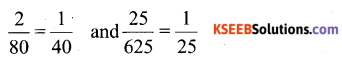No, These are in proportion

d) 200ml : 2.5 litre and Rs 4 : Rs 50
1l = 100 ml
2.5 l = 2500 ml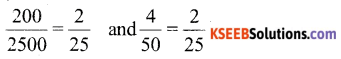Yes , These are in proportion middle terms are 2.5 l, Rs 4
Extreme terms are 200 ml ,Rs 50

error: Content is protected !!